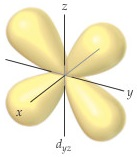# Problem: The accompanying drawing shows a contour plot for a dyz orbital. Consider the quantum numbers that could potentially correspond to this orbital. What is the smallest possible value of the principal quantum number, n?

⚠️Our tutors found the solution shown to be helpful for the problem you're searching for. We don't have the exact solution yet.

###### Problem Details

The accompanying drawing shows a contour plot for a dyz orbital. Consider the quantum numbers that could potentially correspond to this orbital.What is the smallest possible value of the principal quantum number, n?